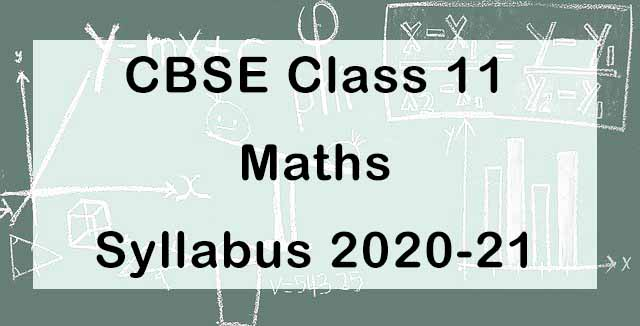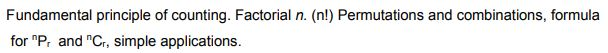## CBSE Class 11 Maths Syllabus 2020-21| Check Latest Exam Pattern

CBSE has released revised Class 11 Maths Syllabus 2020-21. This year, the board has decided to reduce the syllabus by 30 percent.  The annual board examination will be of 80 marks, while internal assessment (Periodic Tests and Mathematics Activities) will be of 20 Marks.No. Units Marks I Sets and Functions 23 II Algebra 30 III Coordinate Geometry 10 IV Calculus 07 V Statistics and Probability 10 - Total 80 - Internal Assessment 20

In Board examination, the listed topics will come. The written examination will be of 80 marks. No chapter/unit-wise weightage is given. There will be no overall choice in the question paper. However, 33% internal choices will be given in all the sections

Unit-I: Sets and Functions

1. Sets

Sets and their representations. Empty set. Finite and Infinite sets. Equal sets. Subsets. Subsets of a set of real numbers especially intervals (with notations). Power set. Universal set. Venn diagrams.
Union and Intersection of sets.

2. Relations & Functions

Ordered pairs. Cartesian product of sets. Number of elements in the Cartesian product of two finite sets. Cartesian product of the set of reals with itself ( R x R only).Definition of relation, pictorial diagrams, domain, co-domain and range of a relation. Function as a special type of relation. Pictorial representation of a function, domain, co-domain and range of a function. Real valued functions, domain and range of these functions, constant, identity, polynomial, rational, modulus, signum, exponential, logarithmic and greatest integer functions, with their graphs.

3. Trigonometric Functions

Positive and negative angles. Measuring angles in radians and in degrees and conversion from one measure to another. Definition of trigonometric functions with the help of unit circle. Truth of the identity sin2x + cos2x = 1, for all x. Signs of trigonometric functions. Domain and range of trigonometric functions and their graphs. Expressing sin (x±y) and cos (x±y) in terms of sinx, siny, cosx & cosy and their simple applications. Deducing identities like the following:Identities related to sin2x, cos2x, tan2 x, sin3x, cos3x and tan3x.

Unit-II: Algebra

1. Complex Numbers and Quadratic Equations

Need for complex numbers, especially√−1, to be motivated by inability to solve some of the quardratic equations. Algebraic properties of complex numbers. Argand plane. Statement of Fundamental Theorem of Algebra, solution of quadratic equations (with real coefficients) in the complex number system.

2. Linear Inequalities

Linear inequalities. Algebraic solutions of linear inequalities in one variable and their representation on the number line. Graphical solution of linear inequalities in two variables. Graphical method of finding a solution of system of linear inequalities in two variables.

3. Permutations and Combinations4. Sequence and Series

Sequence and Series. Arithmetic Progression (A. P.). Arithmetic Mean (A.M.) Geometric Progression (G.P.), general term of a G.P., sum of n terms of a G.P., infinite G.P. and its sum, geometric mean (G.M.), relation between A.M. and G.M.

Unit-III: Coordinate Geometry

1. Straight Lines

Brief recall of two dimensional geometry from earlier classes. Slope of a line and angle between two lines. Various forms of equations of a line: parallel to axis, point -slope form, slope-intercept form, two-point form, intercept form and normal form. General equation of a line. Distance of a point from a line.

2. Conic Sections

Sections of a cone: circles, ellipse, parabola, hyperbola. Standard equations and simple properties of parabola, ellipse and hyperbola. Standard equation of a circle.

3. Introduction to Three-dimensional Geometry

Coordinate axes and coordinate planes in three dimensions. Coordinates of a point. Distance between two points and section formula.

Unit-IV: Calculus

1. Limits and Derivatives

Derivative introduced as rate of change both as that of distance function and geometrically. Intuitive idea of limit. Limits of polynomials and rational functions trigonometric, exponential and logarithmic functions. Definition of derivative relate it to scope of tangent of the curve, derivative of sum, difference, product and quotient of functions. Derivatives of polynomial and trigonometric functions.

Unit-V: Statistics and Probability

1. Statistics

Measures of Dispersion: Range, Mean deviation, variance and standard deviation of ungrouped/grouped data.

2. Probability

Random experiments; outcomes, sample spaces (set representation). Events; occurrence of events, ‘not’, ‘and’ and ‘or’ events, exhaustive events, mutually exclusive events, Probability of an event, probability of ‘not’, ‘and’ and ‘or’ events.

Internal Assessment

 Internal Assessment 20 Marks Periodic Tests ( Best 2 out of 3 tests conducted) 10 Marks Mathematics Activities 10 Marks

Prescribed Books:

1) Mathematics Textbook for Class XI, NCERT Publications